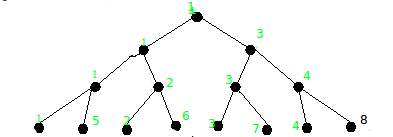# Reconstructing Segment Tree

We are given 2*N – 1 integers. We need to check whether it is possible to construct a Range Minimum Query segment tree for an array of N distinct integers from these integers. If so, we must output the segment tree array. N is given to be a power of 2.

An RMQ segment tree is a binary tree where each node is equal to the minimum value of its children. This type of tree is used to efficiently find the minimum value of elements in a given range.

```Input  : 1 1 1 1 2 2 3 3 3 4 4 5 6 7 8
Output : 1 1 3 1 2 3 4 1 5 2 6 3 7 4 8
The segment tree is shown belowInput  : -381 -460 -381 95 -460 855 -242
405 -460 982 -381 -460 95 981 855
Output : -460 -460 -381 -460 95 -381 855
-460 -242 95 405 -381 981 855 982
By constructing a segment tree from the output,
we can see that it a valid tree for RMQ and the
leaves are all distinct integers.
```

## Recommended: Please try your approach on {IDE} first, before moving on to the solution.

What we first do is iterate through the given integers counting the number of occurrences of each number, then sorting them by value. In C++, we can use the data structure map, which stores elements in sorted order.

Now we maintain a queue for each possible level of the segment tree. We put the initial root of the tree (array index 0) into the queue for the max level. We then insert the smallest element into the leftmost nodes. We then detach these nodes from the main tree. As we detach a node, we create a new tree of height h – 1, where h is the height of the current node. We can see this in figure 2. We insert the root node of this new tree into the appropriate queue based on its height.

We go through each element, getting a tree of appropriate height based on the number of occurrences of that element. If at any point such a tree does not exist, then it is not possible to create a segment tree.

 `// C++ Program to Create RMQ Segment Tree ` `#include ` `using` `namespace` `std; ` ` `  `// Returns true if it is possible to construct ` `// a range minimum segment tree from given array. ` `bool` `createTree(``int` `arr[], ``int` `N) ` `{ ` `    ``// Store the height of the final tree ` `    ``const` `int` `height = log2(N) + 1; ` ` `  `    ``// Container to sort and store occurrences of elements ` `    ``map<``int``, ``int``> multi; ` ` `  `    ``// Insert elements into the container ` `    ``for` `(``int` `i = 0; i < 2 * N - 1; ++i) ` `        ``++multi[arr[i]]; ` ` `  `    ``// Used to store new subtrees created ` `    ``set<``int``> Q[height]; ` ` `  `    ``// Insert root into set ` `    ``Q[height - 1].emplace(0); ` ` `  `    ``// Iterate through each unique element in set ` `    ``for` `(map<``int``, ``int``>::iterator it = multi.begin(); ` `        ``it != multi.end(); ++it) ` `    ``{ ` `        ``// Number of occurrences is greater than height ` `        ``// Or, no subtree exists that can accomodate it ` `        ``if` `(it->second > height || Q[it->second - 1].empty()) ` `            ``return` `false``; ` ` `  `        ``// Get the appropriate subtree ` `        ``int` `node = *Q[it->second - 1].begin(); ` ` `  `        ``// Delete the subtree we grabbed ` `        ``Q[it->second - 1].erase(Q[it->second - 1].begin()); ` ` `  `        ``int` `level = 1; ` `        ``for` `(``int` `i = node; i < 2 * N - 1; ` `            ``i = 2 * i + 1, ++level) ` `        ``{ ` `            ``// Insert new subtree created into root ` `            ``if` `(2 * i + 2 < 2 * N - 1) ` `                ``Q[it->second - level - 1].emplace(2 * i + 2); ` ` `  `            ``// Insert element into array at position ` `            ``arr[i] = it->first; ` `        ``} ` `    ``} ` `    ``return` `true``; ` `} ` ` `  `// Driver program ` `int` `main() ` `{ ` `    ``int` `N = 8; ` `    ``int` `arr[2 * N - 1] = {1, 1, 1, 1, 2, 2, ` `                 ``3, 3, 3, 4, 4, 5, 6, 7, 8}; ` `    ``if` `(createTree(arr, N)) ` `    ``{ ` `        ``cout << ``"YES\n"``; ` `        ``for` `(``int` `i = 0; i < 2 * N - 1; ++i) ` `            ``cout << arr[i] << ``" "``; ` `    ``} ` `    ``else` `        ``cout << ``"NO\n"``; ` `    ``return` `0; ` `} `

Output:

```YES
1 1 3 1 2 3 4 1 5 2 6 3 7 4 8
```

Main time complexity is caused by sorting elements.
Time Complexity: O(N log N)
Space Complexity: O(N)